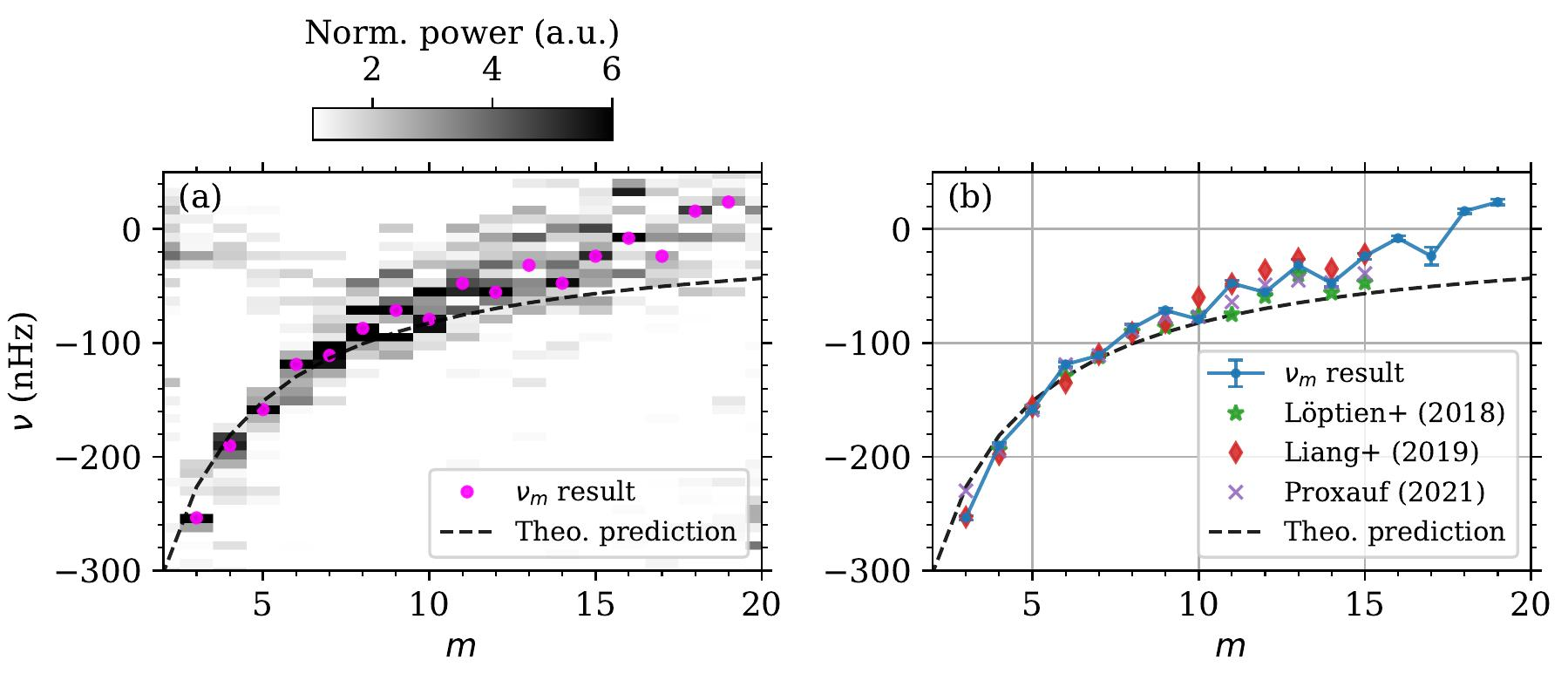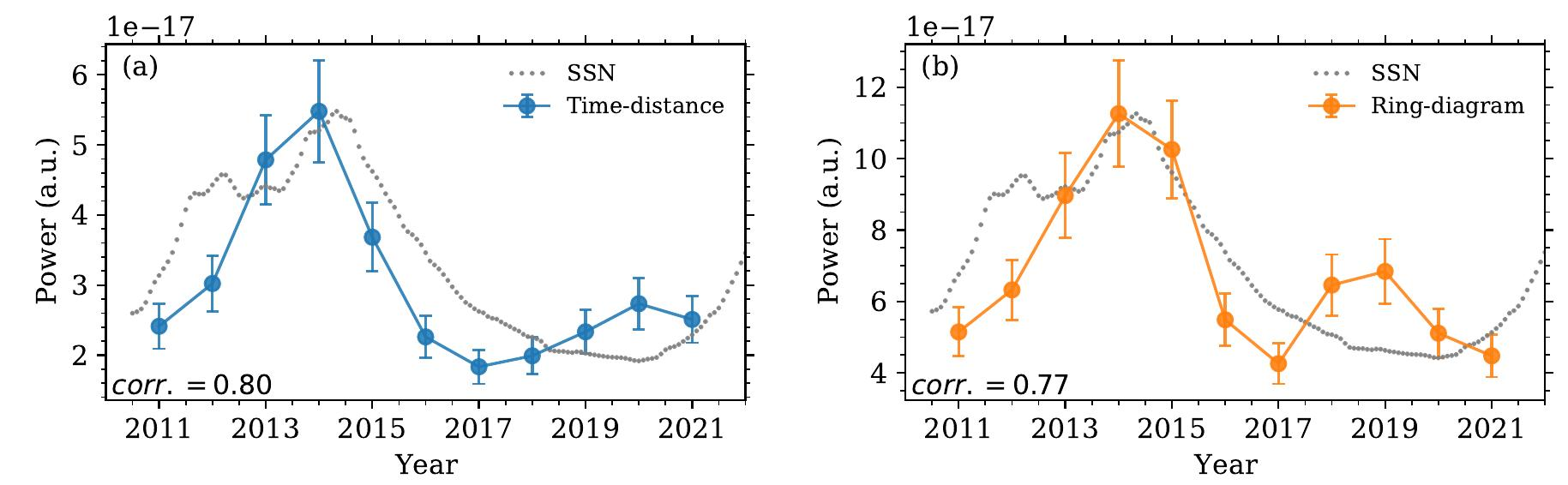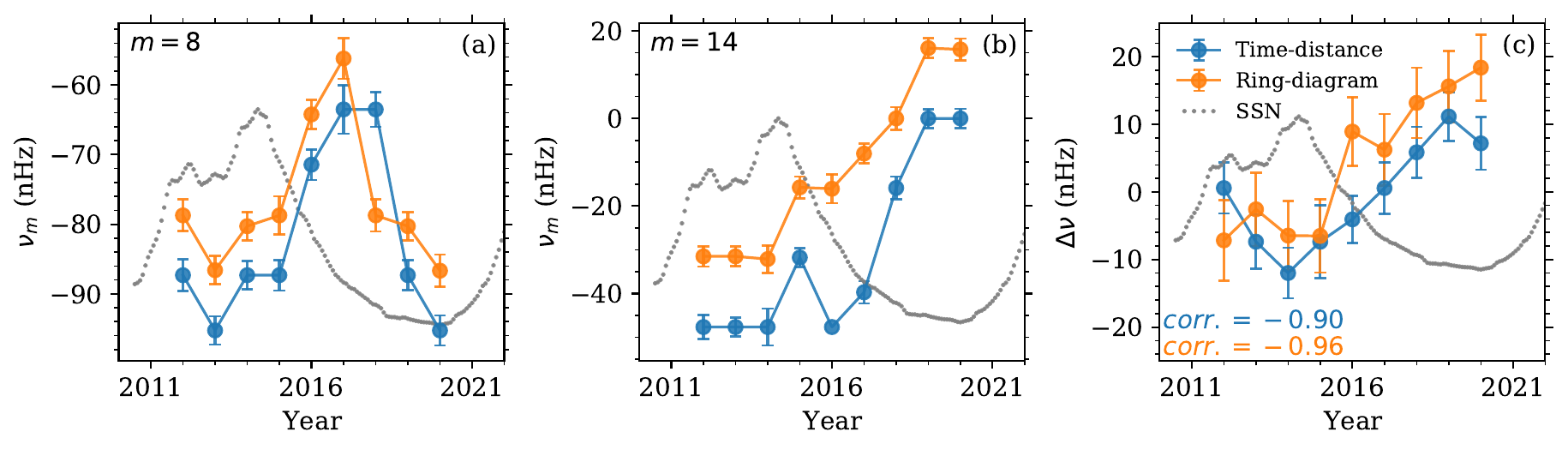# 196. Observations of Rossby wave parameter variations during solar cycle 24

Contributed by Mattias Waidele. Posted on September 25, 2023

Mattias Waidele & Junwei Zhao
W. W. Hansen Experimental Physics Laboratory, Stanford University, Stanford, CA 94305-4085, USA

Rossby waves are global scale waves with frequencies similar to the solar rotation rate. They describe vortical gas motions at low latitudes propagating retrograde. Although Rossby waves can be observed in Earth’s atmosphere and have been predicted in stellar atmospheres, they were detected only recently. Measuring Rossby wave parameters may further the understanding of angular momentum transport and the modulation of magnetic fields in the Sun.

Using the SDO time–distance pipeline horizontal subsurface flow fields, we measure Rossby wave power pm up to an azimuthal degree of m = 19 (see Figure 1). This is larger than what is achievable by the commonly used 15°–tile ring–diagram data. The resulting mode frequencies νm appear close to the dispersion relation expected theoretically and show the so far poorly–understood deviation towards larger values at m > 10.Figure 1| Power pm and frequency νm results from 12 years of time–distance subsurface flows. (a) Normalized power pm with νm results overplotted for (magenta dots). The power is normalized for all m individually to the average power within ν ∈ [−300, 50] nHz. The theoretical expectation for νm is shown as a black dashed curve. (b) Comparison of νm results from this work with those from selected studies [1, 3, 4].

The data cover a total of 12 years, allowing us to perform a time–dependent investigation of mode parameters, i.e. the power amplitude pm as well as the mode frequencies νm. By dividing the full period into 2 year segments with 1 year overlap, we can study pm and νm during the progression of solar cycle 24. For the power amplitude, this is done by integrating all power between 3 ≤ m ≤ 16 around the detected mode frequencies, resulting in a time–dependent power curve with 1 data point per year. A striking correlation to the solar activity (indicated by the monthly smoothed sunspot number, SSN) can be seen in Figure 2a. Performing the same analysis on ring–diagram data, a similar behavior is seen in Figure 2b.Figure 2| Average Rossby wave power as a function of time. (a) Comparison of average wave power with the monthly smoothed sunspot number (SSN, normalized to fit the scale). The value corr. denotes the sample correlation coefficient between the wave power and the SSN. (b) Same as panel (a), but for the ring–diagram data.

The time–dependent frequency locations νm can be studied in a similar fashion, although 3-year segments are needed for more precision. Although the mode frequencies also show a clear time–dependence, the overall behavior is more complex. This can be seen by comparing m = 8 (Figure 3a) and m = 14 (Figure 3b), where modes tend to move faster (in retrograde direction) during the cycle maximum before slowing as the cycle declines, but go back to moving faster again in the cycle minimum for m = 8. For an overall picture, we average the deviation of νm from a reference νref, where νref correspond to the full 12-year period shown in Figure 1a. The result, ∆ν = Σmm − νref) /13 is shown in Figure 3c.Figure 3| Frequency locations νm (for (a) m = 8 and (b) m = 14) and average frequency deviation ∆ν (panel c) shown as functions of time for time–distance data (blue) and ring–diagram data (orange). Similar to Figure 3, the monthly SSN is shown as gray dots.

Overall, we observe a correlation between Rossby wave parameters and the solar cycle. While the power amplitude shows a positive correlation, the average frequency deviation ∆ν is anti–correlated, indicating a larger retrograde phase speed during solar maximum. The underlying mechanism behind this observation has not been studied and it is therefore unclear if Rossby waves interact directly with the solar magnetic field, or if the interaction happens indirectly through large scale flows or the excitation of Rossby waves.

Regardless, these observations open up a new study topic to further our understanding of the intricacies of the solar cycle. For the full details of this work, please refer to our recent publication .

### References

 Löptien, B., Gizon, L., Birch, A. C., et al. 2018, NatAs, 2, 568
 Zhao, J., Couvidat, S., Bogart, R. S., et al. 2012, SoPh, 275, 375
 Liang, Z.-C., Gizon, L., Birch, A. C., & Duvall, T. L. 2019, A&A, 626, A3
 Proxauf, B. 2021, PhD Thesis, Univ. of Göttingen
 Waidele, M. & Zhao, J., 2023, ApJL, 954, L26